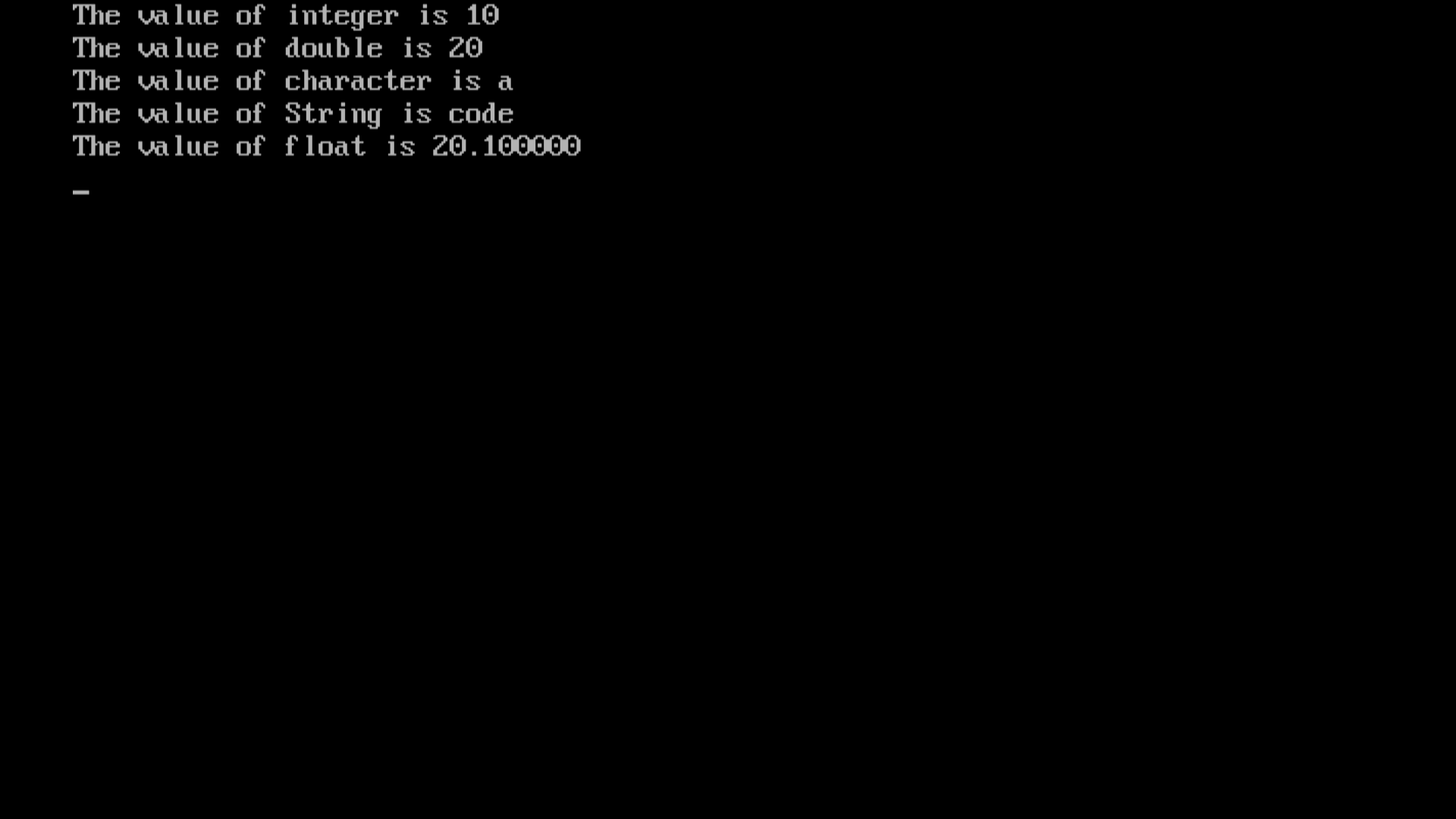Article

# Chapter-5 (format specifier in c)

In C, format specifiers are used to specify the type of data being read or displayed when using functions like printf and scanf. These are a few frequently used C format specifiers:

Format Specifier:-

%d: Integer

%lg: double

%f: Float

%c: Character

%s: String

%p: Pointer

%x, %X: Hexadecimal (lowercase or uppercase)

%o: Octal

%u: Unsigned Integer

%e, %E: Scientific notation (lowercase or uppercase)

%g, %G: Uses %f or %e based on the value

%%: Percent sign

Here some of the format specifiers used in the below example in C:-

Example:-

#include<stdio.h>

#include<conio.h>

void main()

{

int a=10;double b=20;char c='a';char d="code";float f=20.10;

clrscr();

printf("The value of integer is %d\n",a);

printf("The value of double is %lg\n",b);

printf("The value of character is %c\n",c);

printf("The value of String is %s\n",d);

printf("The value of float is %f\n",f);

getch();

}